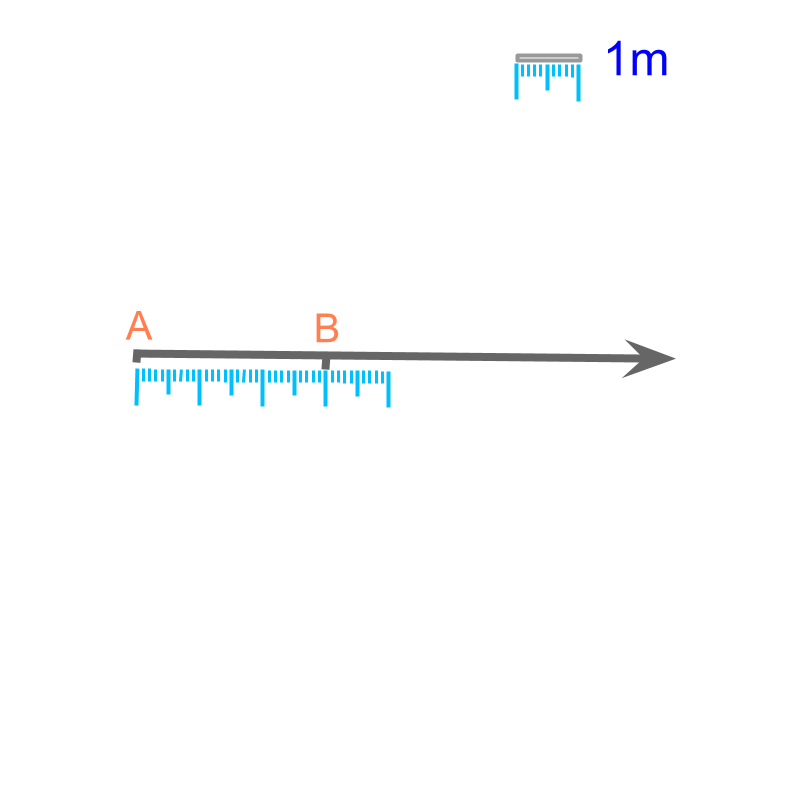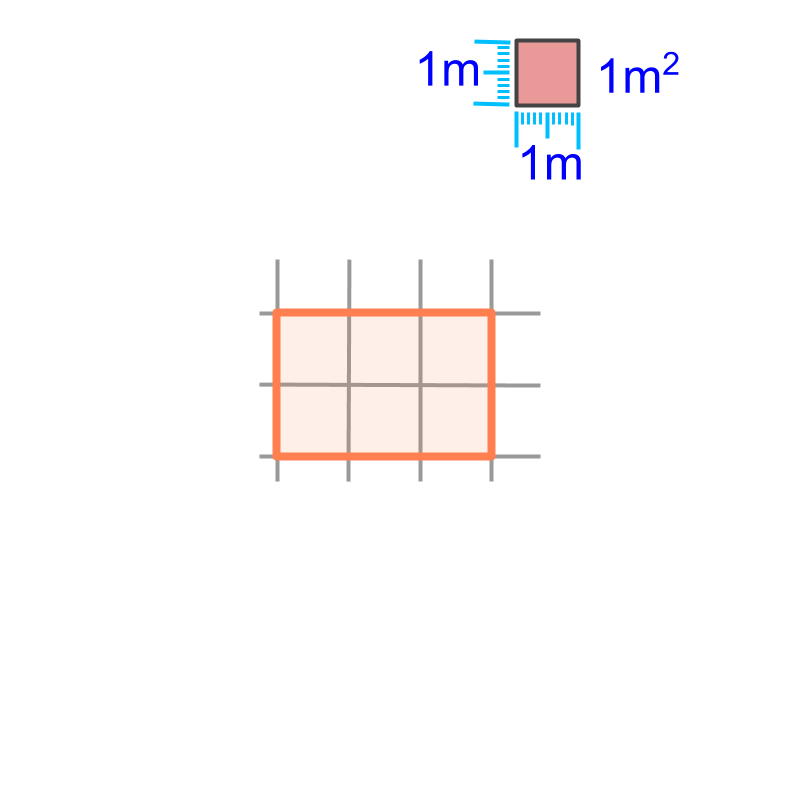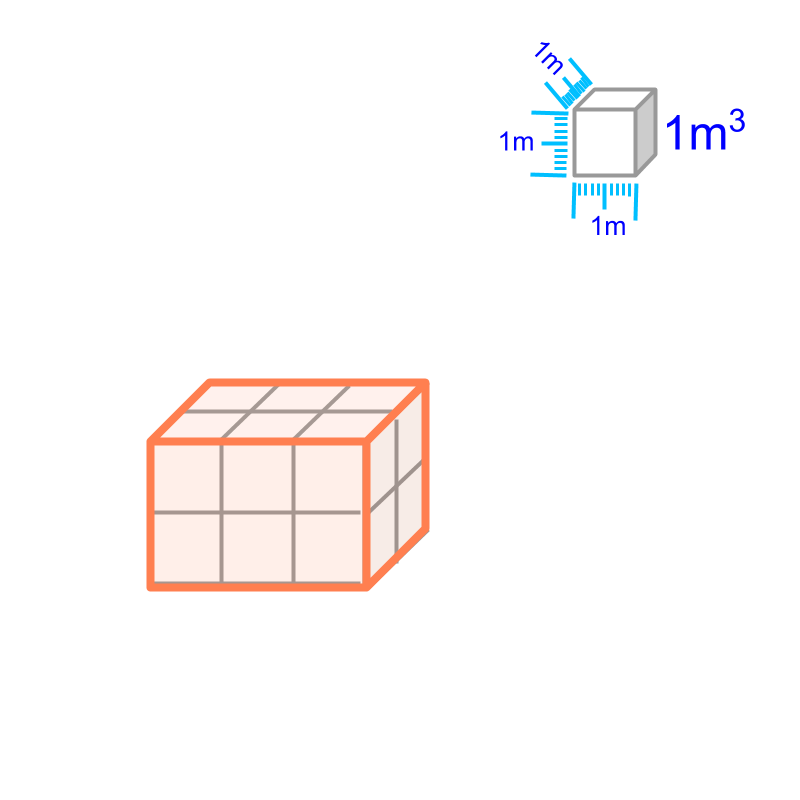maths > mensuration-high

Summary of Measurement Basics

what you'll learn...

Overview

Revising the concepts:

•   Absolute Standards are standards defined independent of any other measures.

•   Derived Standards are standards derived using one or more of absolute standards.

•   Length is the distance-span between two points.

•   Area is the surface-span of a closed plane figure.

•   Volume is the space-span of a closed solid figure.

standard measure of length

Meter is a standard unit to measure length.

The unit of length "meter" is specified in reference to the standard provided by the International System of Units.

A metal-bar of specific material and at specific temperature is kept in International Bureau of Weights and Measures. The distance between two markings on that bar is defined as the standard unit meter.

The definition of meter is also given in reference to distances that do not change and can be calculated or measured. For example, the distance between equator and north pole along a specific meridian is a constant. One ten-millionth of that distance is defined as one meter.

Another example is : Speed of light in vacuum is constant. So the distance traveled by light in vacuum in one in 300,000,000 seconds is one meter.

absolute & derived

It is noted that the meter is defined independent of any other measures.

Meter is an example of an absolute standard. The word absolute means "existing independent and not relative to other". That is, the standard measure "meter" is defined independent of other measures.

To understand absolute standards better, some examples for absolute standards are provided

•  The unit of temperature, degree Celsius is defined based on: melting point of ice is ${0}^{\circ }C$${0}^{\circ} C$ and boiling point of water is ${100}^{\circ }C$${100}^{\circ} C$.

•  The unit of time, second is defined based on: A normal day consists of $24$$24$ hours with $60$$60$ minutes in an hour and $60$$60$ seconds in a minute.

Comparing the above with the units that are not independent

•  The unit of area, square meter, is derived from unit of length meter.

•  The unit of speed, meter per second, is derived from units of distance (meter) and time (second).

Such standards are called "derived standards".

The word "absolute" means: existing independent and not relative to other. The word "absolute" is from a root word meaning free and unrestricted.

The word "derived" means: dependent and in reference to other. The word "derived" is from root word meaning to draw out and take from.

Absolute Standards : An unit of measurement, widely accepted and used in reference to a standardized prototype.

Derived Standards : An unit of measurement that is defined using other absolute standards.

absolute & derived

Length : The distance-span between two points is the length. It is measured in meter or in one of its other forms.Length is specified as a number in reference to the reference-prototype-standard meter (or in one of other derived or similar forms).

Area of a plane figure : The surface-span of a plane figure is the area of the surface. It is measured in square meter (or in one of other derived or similar forms).Area is specified as a number in reference to the surface-span of a square of $1$$1$ meter side.

Volume of a solid : The space-span of a solid is the volume of the solid. It is measured in cubic meter (or in one of other derived or similar forms.)Volume is specified as a number in reference to the space-span of a cube of $1$$1$ meter side.

summary

Revising the concepts:

•   Absolute Standards are standards defined independent of any other measures.

•   Derived Standards are standards derived using one or more of absolute standards.

•   Length is the distance-span between two points.

•   Area is the surface-span of a closed plane figure.

•   Volume is the space-span of a closed solid figure.

Outline